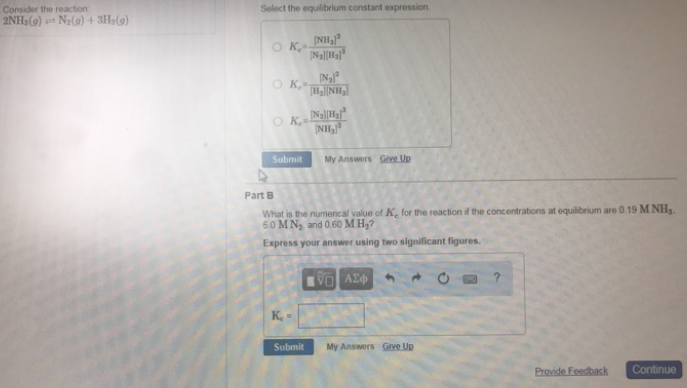# Problem: Consider the reaction: 2 NH3(g) ⇌ N2(g) + 3 H2(g) Select the equilibrium constant expression. (A) Kc = [NH3]2/[N2][H2]3 (B) Kc = [N2]2/[H2][NH3] (C) Kc = [N2][H2]3/[NH3]2 What is the numerical value of Kc for the reaction if the concentrations at equilibrium are 0.19 M NH3, 5.0 M N2, and 0.60 M H2? Express your answer using two significant figures.

###### FREE Expert Solution
84% (67 ratings)###### Problem Details

Consider the reaction:

2 NH3(g) ⇌ N2(g) + 3 H2(g)

Select the equilibrium constant expression.

(A) Kc = [NH3]2/[N2][H2]3

(B) Kc = [N2]2/[H2][NH3

(C) Kc = [N2][H2]3/[NH3]2

What is the numerical value of Kc for the reaction if the concentrations at equilibrium are 0.19 M NH3, 5.0 M N2, and 0.60 M H2